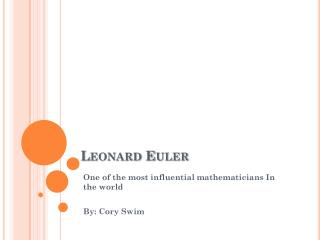DownloadDownload PresentationLeonard Euler

# Leonard Euler

Download Presentation## Leonard Euler

- - - - - - - - - - - - - - - - - - - - - - - - - - - E N D - - - - - - - - - - - - - - - - - - - - - - - - - - -
##### Presentation Transcript

1. Leonard Euler One of the most influential mathematicians In the world By: Cory Swim

2. His life • Born in Basel, Switzerland • Family moved to Riehen when he was one year old, however he moved back and lived with his grandmother at 13 years old. • His school was poor so he never truly learned any mathematics when he was attending. His true math skill were learned in the University of Basel

3. • He had a phenomenal memory which aided him when he went blind, enabling him to publish his works by dictation. • His father was preparing him to be a minister but he chose to be a mathematician instead once he reached the University of Basel • He won the coveted annual award 12 times in his career • He published more papers than any other mathematician in history

4. His accomplishments: • He created the Euler Method (Go Figure) • He established the greek letter pi to denote the diameter of a circle • He established many other notations such as “e” for the natural base of a logarithm, “i” for the square root of negative one, and F(x) to represent a function. • He was the first to truly study sine and cosine functions deeply

5. Euler Equations • This special case shown above is known as Euler’s Identity • Euler-Mascheroni Constant • Proof that the sum of the reciprocals of the primes diverges

6. More on the before equations

7. Other fun Euler Stuff Euler’s line is the red one! Euler circle is… the circle! -->

8. Seven Bridges of Königsberg. In 1736 Euler solved the problem known as “Seven bridges of Konigsburg” The city of Konigsberg , Prussia was set on the PregelRiver, and included two large islands which were connected to each other and the mainland by seven bridges. The problem is to decide whether it is possible to follow a path that crosses each bridge exactly once and returns to the starting point. It is not: there is no Eulerian circuit. Euler also discovered the formula V − E + F = 2 relating the number of vertices, edges, and faces of a convex polyhedron, and hence of a planar graph. The constant in this formula is now known as the Euler characteristic for the graph (or other mathematical object), and is related to the genus of the object

9. The Euler Method • A way to approximate what a curve will look like using it’s derivative without finding the antiderivative

10. Blue Line: The Euler Method • Green Line: The Midpoint Method • Red Line: the exact true equation (Which happens to be y = e^t) with step size of h=1

11. How to use the Euler Method • A0 , the starting point, is known so that we can use the derivative function to find the slope. • If we pretend A1 is still on the curve the same reasoning as for point A0 can be used. • After these steps are taken for A0, A1, A2, etc… a graph can be pieced together using the tangent lines(see graph). • The Euler Method line does not diverge far from the actual line

12. Resources • Scott, Simon. “A Math Great Gets His Due”, Weekend Edition Saturday; 2007. • Leonard Euler (1707-1783) May 23, 2007 http://www-groups.dcs.st-and.ac.uk/~history/Mathematicians/Euler.html • Wikipedia, the free encyclopedia May 23, 2007 http://en.wikipedia.org/wiki/Leonhard_Euler • Mr. Sidanycz